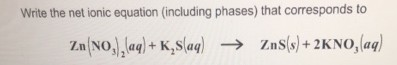# Problem: Write the net ionic equation (including phases) that corresponds to Zn(NO3)2(aq) + K2S(aq) → ZnS(s) + 2KNO3(aq).

###### FREE Expert Solution

In a net ionic equation the spectator ions are removed and what remains is the net ionic equation

100% (359 ratings)###### Problem Details

Write the net ionic equation (including phases) that corresponds to

Zn(NO3)2(aq) + K2S(aq) → ZnS(s) + 2KNO3(aq).